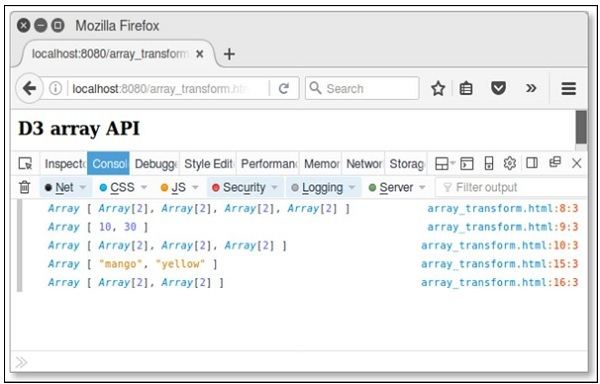# D3.js - 数组 API

D3 包含一组模块。你可以单独使用每个模块，也可以将模块集合一起使用以执行操作。本章详细介绍了 Array API。

## 什么是数组？

Array 包含相同类型的固定大小的顺序元素集合。数组用于存储数据集合，但将数组视为相同类型的变量集合通常更有用。

## 配置 API

<script src = "https://d3js.org/d3-array.v1.min.js"></script>
<body>
<script>
</script>
</body>


## 数组统计 API 方法

• d3.min(array)
• d3.max(array)
• d3.extent(array)
• d3.sum(array)
• d3.mean(array)
• d3.quantile(array)
• d3.variance(array)
• d3.deviation(array)

### d3.min(array)

<script>
var data = [20,40,60,80,100];
console.log(d3.min(data));
</script>


### d3.max(array)

<script>
var data = [20,40,60,80,100];
console.log(d3.max(data));
</script>


### d3.extent(array)

<script>
var data = [20,40,60,80,100];
console.log(d3.max(data));
</script>


### d3.sum(array)

<script>
var data = [20,40,60,80,100];
console.log(d3.sum(data));
</script>


### d3.mean(array)

<script>
var data = [20,40,60,80,100];
console.log(d3.mean(data));
</script>


### d3.quantile(array)

var data = [20, 40, 60, 80, 100];
d3.quantile(data, 0); // output is 20
d3.quantile(data, 0.5); // output is 60
d3.quantile(data, 1); // output is 100


### d3.variance(array)

<script>
var data = [20,40,60,80,100];
console.log(d3.variance(data));
</script>


### d3.deviation(array)

<script>
var data = [20,40,60,80,100];
console.log(d3.deviation(data));
</script>


<html>
<script type = "text/javascript" src = "https://d3js.org/d3.v4.min.js"></script>

<body>
<h3>D3 array API</h3>
<script>
var data = [20,40,60,80,100];
console.log(d3.min(data));
console.log(d3.max(data));
console.log(d3.extent(data));
console.log(d3.sum(data));
console.log(d3.mean(data));
console.log(d3.quantile(data,0.5));
console.log(d3.variance(data));
console.log(d3.deviation(data));
</script>
</body>
</html>## 数组搜索 API 方法

• d3.scan(array)
• d3.ascending(a，b)

### d3.scan(array)

var array = [{one: 1}, {one: 10}];
console.log(d3.scan(array, function(a, b) { return a.one - b.one; })); // output is 0
console.log(d3.scan(array, function(a, b) { return b.one - a.one; })); // output is 1


### d3.ascending(a, b)

function ascending(a, b) {
return a < b ? -1 : a > b ? 1 : a > =  b ? 0 : NaN;
}


<html>
<script type = "text/javascript" src = "https://d3js.org/d3.v4.min.js"></script>

<body>
<h3>D3 array API</h3>
<script>
var array = [{one: 1}, {one: 10}];
console.log(d3.scan(array, function(a, b) { return a.one - b.one; })); // 0
console.log(d3.scan(array, function(a, b) { return b.one - a.one; })); // 1
</script>
</body>
</html>


## 数组转换 API

• d3.cross(a，b [, reducer])
• d3.merge(array)
• d3.pairs(array [，reducer])
• d3.permute(array, index)
• d3.zip(array)

### d3.cross(a，b [, reducer])

d3.cross([10, 20], ["a", "b"]); // output is [[10, "a"], [10, "b"], [20, "a"], [20, "b"]]


### d3.merge(array)

d3.merge([, ]); // output is [10, 20]


### d3.pairs(array [，reducer])

d3.pairs([10, 20, 30, 40]); // output is [[10, 20], [20, 30], [30, 40]]


### d3.permute(array, index)

var object = {fruit:"mango", color: "yellow"},
fields = ["fruit", "color"];
d3.permute(object, fields); // output is "mango" "yellow"


### d3.zip(array)

d3.zip([10, 20], [30, 40]); // output is [[10, 30], [20, 40]]


<html>
<script type = "text/javascript" src = "https://d3js.org/d3.v4.min.js"></script>

<body>
<h3>D3 array API</h3>
<script>
console.log(d3.cross([10, 20], ["a", "b"]));
console.log(d3.merge([, ]));
console.log(d3.pairs([10, 20, 30, 40]));
var object = {fruit:"mango", color: "yellow"},
fields = ["fruit", "color"];
console.log(d3.permute(object, fields));
console.log(d3.zip([10, 20], [30, 40]));
</script>
</body>
</html>